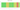# Nfk

## Eritrean Nakfa

#### how has the value of the currency changed in the last year?## USD 1 = ERN 15.001

How much is10 US dollars worth inEritrean nakfas?
At the current exchange rate, 10 US dollars is worth 150.01 Eritrean nakfas
How much is50 US dollars worth inEritrean nakfas?
At the current exchange rate, 50 US dollars is worth 750.03 Eritrean nakfas
How much is100 US dollars worth inEritrean nakfas?
At the current exchange rate, 100 US dollars is worth 1,500.06 Eritrean nakfas
How much is500 US dollars worth inEritrean nakfas?
At the current exchange rate, 500 US dollars is worth 7,500.31 Eritrean nakfas
How much is2,000 US dollars worth inEritrean nakfas?
At the current exchange rate, 2,000 US dollars is worth 30,001.24 Eritrean nakfas
How much is10 US dollars worth inEritrean nakfas?
At the current exchange rate, 10 US dollars is worth 150.01 Eritrean nakfas
How much is50 US dollars worth inEritrean nakfas?
At the current exchange rate, 50 US dollars is worth 750.03 Eritrean nakfas
How much is100 US dollars worth inEritrean nakfas?
At the current exchange rate, 100 US dollars is worth 1,500.06 Eritrean nakfas
How much is500 US dollars worth inEritrean nakfas?
At the current exchange rate, 500 US dollars is worth 7,500.31 Eritrean nakfas
How much is2,000 US dollars worth inEritrean nakfas?
At the current exchange rate, 2,000 US dollars is worth 30,001.24 Eritrean nakfas